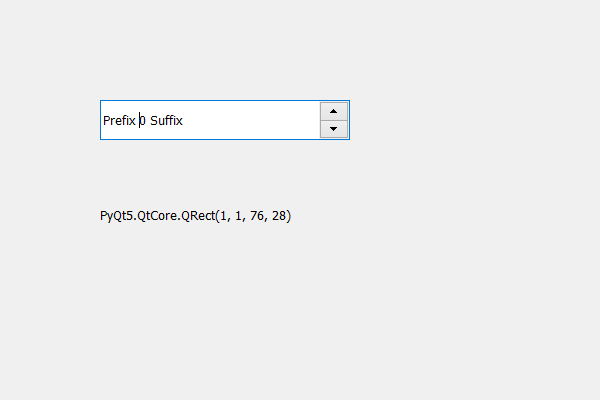GFG App
Open AppBrowser
Continue

# PyQt5 QSpinBox – Getting Bounding Rectangle from the Children Region

In this article we will see how we can get the bounding rectangle from the children region of spin box, children region holds the combined region occupied by the spin box’s children. In order to get the children region we use `childrenRegion` method. Bounding rectangle means the rectangle which will cover i.e bound the whole children region.

In order to do this we use `boundingRect` method with the children region object of the spin box

Syntax : children_region.boundingRect()

Argument : It takes no argument

Return : It returns QRect object

Below is the implementation

 `# importing libraries ` `from` `PyQt5.QtWidgets ``import` `*`  `from` `PyQt5 ``import` `QtCore, QtGui ` `from` `PyQt5.QtGui ``import` `*`  `from` `PyQt5.QtCore ``import` `*`  `import` `sys ` ` `  ` `  `class` `Window(QMainWindow): ` ` `  `    ``def` `__init__(``self``): ` `        ``super``().__init__() ` ` `  `        ``# setting title ` `        ``self``.setWindowTitle(``"Python "``) ` ` `  `        ``# setting geometry ` `        ``self``.setGeometry(``100``, ``100``, ``600``, ``400``) ` ` `  `        ``# calling method ` `        ``self``.UiComponents() ` ` `  `        ``# showing all the widgets ` `        ``self``.show() ` ` `  `        ``# method for widgets ` `    ``def` `UiComponents(``self``): ` `        ``# creating spin box ` `        ``self``.spin ``=` `QSpinBox(``self``) ` ` `  `        ``# setting geometry to spin box ` `        ``self``.spin.setGeometry(``100``, ``100``, ``250``, ``40``) ` ` `  `        ``# setting range to the spin box ` `        ``self``.spin.setRange(``0``, ``999999``) ` ` `  `        ``# setting prefix to spin ` `        ``self``.spin.setPrefix(``"Prefix "``) ` ` `  `        ``# setting suffix to spin ` `        ``self``.spin.setSuffix(``" Suffix"``) ` ` `  `        ``# getting the children region ` `        ``children_region ``=` `self``.spin.childrenRegion() ` ` `  `        ``# creating a label ` `        ``label ``=` `QLabel(``self``) ` ` `  `        ``# setting geometry to the label ` `        ``label.setGeometry(``100``, ``200``, ``200``, ``30``) ` ` `  `        ``# getting bounding rectangle from the children region ` `        ``bounding_rectangle ``=` `children_region.boundingRect() ` ` `  `        ``# setting text to the label ` `        ``label.setText(``str``(bounding_rectangle)) ` ` `  ` `  ` `  `# create pyqt5 app ` `App ``=` `QApplication(sys.argv) ` ` `  `# create the instance of our Window ` `window ``=` `Window() ` `window.spin.setFocus() ` `# start the app ` `sys.exit(App.``exec``()) `

Output :My Personal Notes arrow_drop_up